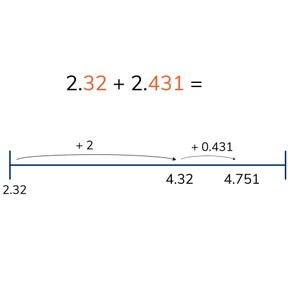Addition with decimal numbers with 1, 2, or 3 decimal places

# Addition with decimal numbers with 1, 2, or 3 decimal places

No account needed.8,000 schools use Gynzy92,000 teachers use Gynzy1,600,000 students use Gynzy

## General

Students learn to add decimal numbers with 1, 2, and 3 decimals. They also learn to determine which numbers are important in story problems.

6.NS.B.3

## Relevance

Discuss with students that it is important that they are able to calculate problems with decimal numbers with 1, 2, and 3 decimal places so they can calculate, for example, how long something takes.

## Introduction

Students practice with different addition problems with decimal numbers. They practice the following combinations: adding with 1 decimal place, with 2 decimal places, and with 1 and 2 decimal places.

## Development

Next students are given addition problems in story form. Tell the students which steps they should take in solving a story problem and do one as a class. Then have students solve a story problem. Ask them which steps they took to solve the problem.

Check that students are able to add decimal numbers with 1, 2, and 3 decimal places by asking the following questions:
- Which methods can you use to add decimal numbers with 1, 2, and 3 decimal places?
- Which method do you like best?
- How do you solve a story problem?
- Which steps do you use?

## Guided practice

Students first practice adding decimal numbers with 1, 2, and 3 decimal places. They are then given a story problem and must determine the relevant numbers and use them to solve for the answer.

## Closing

Check that students are able to tell you which methods they can use to add decimal numbers with 1,2, and 3 decimal places. Then ask how they would solve a story problem. Check with a few exercises as a class that students are able to solve these addition problems. Emphasize that students should choose the method they like best. You can challenge the class with the problems hidden under "Extra" these are extra challenging addition problems.,
For the final exercise, have students race against the timer, by dragging the decimal points to the correct place. You can make it more or less challenging by modifying the time available.

## Teaching tips

Students who have difficulty adding decimal numbers with 1,2, and 3 decimals can first practice placing decimal numbers on the number line. Give them a decimal number with 1 decimal place and have them add 0.1. Then practice with 2 decimal places, and finally 3 decimal places. They might also be helped by using a Decimal Place Value Chart to help determine which value is added where.

## Instruction materials

Number line
Decimal Place Value Chart

### The online teaching platform for interactive whiteboards and displays in schools

• Save time building lessons

• Manage the classroom more efficiently

• Increase student engagement